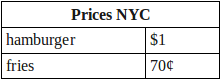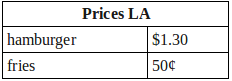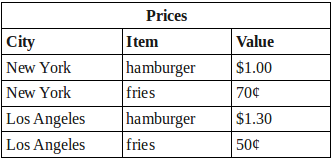# Introduction to PHP Arrays

Many things that make the world as we know it possible are so commonplace that we forget they even exist. One of my favorite examples is the elevator; the idea of a lifting platform or box is very old but it had to wait for the Industrial Revolution to become a practical device. When Mr. Otis installed his first successful elevator in the brand-new E. V. Haughwout Building in 1857, he opened the path for the vertical growth of metropolises like New York City and São Paulo.

Another favorite invention of mine are tables. Not of the dining sort, though they too are immensely important both for eating and working, rather the type that stores information. Such tables are part of our daily life in hundreds of different variations. In many countries, milk, butter and cereal containers display a table of nutritional values – though I wonder who reads and understands them. Most papers, for those that still read them in print, are organized as a sort of table. Many cities, especially in the New World (herein extended to include Australia and New Zealand), are organized as a table with streets as rows and avenues as columns. Children start out with tables as soon as they begin to learn multiplication and divisions; in fact, Portuguese has an interesting word for multiplication tables: taboada, roughly, “a thing put on a table!” Engineering is unthinkable without tables, and tables are the backbones of management techniques like Operational Research and Game Theory.

## What are Arrays?

Tables organize data in such a way that we can easily find correlations or perform straightforward computations. An array is essentially a way to organize data in a table-like manner. The name “array” comes from the same Latin roots as the word “arrangement.”

To help us understand arrays better, let’s begin with a table called Prices NYC to show the price of a hamburger in New York City is one dollar:We can write it as an array called, you’d never guess, `pricesNYC`:

`pricesNYC ("hamburger"=1)`

That piece of information is so simple that representing it on a table or an array seems a waste of time. But what if we add the price of a side of fries? We could write:and:

`pricesNYC ("hamburger"=1, "fries"=.70)`

This is an associative array. In an associative array, each and every value is identified by a label, or sequence of labels, called a key. hamburger and fries are the keys in this example, and their values are 1 and .70 respectively. Keys can also be a numerical sequence instead of labels. In such cases the array is said to be non-associative. Our example as a non-associative array would be:

`pricesNYC (0=1, 1=.70)`

The value at index (or key) 0 is 1 and the value at index 1 is .70. (In most programming languages, non-associative arrays start with an index of 0 for largely historical and mathematically convenient reasons.)

Sometimes the indexes are not explicitly given but the values are still accessible by their position.

`pricesNYC (1, .70)`

Sticking with the associative array, we could create a second table and array if we want to show the same prices for Los Angeles:`pricesLA ("hamburger"=1.30, "fries"=.50)`

Alternatively, we could combine the data from the two tables together like this:```prices ("New York"=pricesNYC ("hamburger"=1, "fries"=.70),
"Los Angeles"=pricesLA ("hamburger"=1.30, "fries"=.50))```

We’ve begin to create arrays within arrays and each value is now identified by the sequence of labels. For example, the key for the price of a burger in NYC is now New York, hamburger. In `prices`, the top-level array has two keys, New York and Los Angeles. Each of these keys point to values that are actually other arrays. These second-level arrays have the labels hamburger and fries which finally point to a number which is the desired price.

In a non-associative fashion, the array would look something like this:

```prices (0=pricesNYC (0=1, 1=.70),
1=pricesLA (0=1.30, 1=.50))```

The price of a burger in NYC is found here with the index 0 0.

## Creating Arrays

Now that the concept of arrays is established, let’s move on to discussing how PHP implements them and, more importantly, how we can create them. The most orthodox method of creating an array is to use the `array()` construct:

``````<?php
\$pricesNYC = array();``````

Our array as created is empty. We can then assign values to the array using the key specified in brackets:

``````<?php
\$pricesNYC["hamburger"] = 1;
\$pricesNYC["fried"] = .70;``````

Alternatively we could have populated the array at the same time we create it using the syntax key `=>` value. The key/value pairs are separated by comma:

``````<?php
\$pricesNYC = array("hamburger" => 1, "fries" => .70);``````

When I was first learning PHP, I often had to go back to the documentation to check whether the correct operator was `=>` or `->`. One day I came up with the mnemonic “this key will be equal to that value” and now I easily remember `=>` is the correct choice.

So what do the above examples look like for non-associative arrays?

``````<?php
\$pricesNYC = array();
\$pricesNYC = 1;
\$pricesNYC = .70;``````

and:

``````<?php
\$pricesNYC = array(0 => 1, 1 => .70);``````

If all you want is a non-associative array, it isn’t necessary to specify the index. PHP automatically uses the next integer value in the key sequence, starting at 0, for key if you do not provide one. These examples produce identical non-associative arrays to those we just saw:

``````<?php
\$pricesNYC = array();
\$pricesNYC[] = 1;
\$pricesNYC[] = .70;``````

and:

``````<?php
\$pricesNYC = array(1, .70);``````

PHP arrays can have mixed keys, that is, some keys can be strings and other can be indexes. So far we’ve seen keys that were either strings (like hamburger) and integers (0).

Now let’s make things a little more interesting by transforming the two-city table into an array. The creation of the empty array is, as before, straightforward:

``````<?php
\$prices = array();``````

Also straightforward is how the array can be populated with the brackets syntax:

``````<?php
\$prices["New York"]["hamburger"] = 1;
\$prices["New York"]["fries"] = 0.70;
\$prices["Los Angeles"]["hamburger"] = 1.30;
\$prices["Los Angeles"]["fries"] = 0.50;``````

or, for non-associative arrays:

``````<?php
\$prices=1;
\$prices=0.7;
\$prices=1.3;
\$prices=0.5;``````

What about assigning all the values when we create the array?
Since our table has two levels, the first being the city and the second one the item, we declare the array like a series of arrays within another:

``````<?php
\$prices = array("Los Angeles" => array("hamburger" => 1.3, "fries" => 0.5),
"New York" => array("hamburger" => 1, "fries" => 0.7));``````

You’ll find you often represent the keys with variables when you work with arrays. This is very useful for creating arrays whose values can be mechanically computed. For example, this loop creates an 10-element array with values that are the square of the keys:

``````<?php
\$squares = array();
for (\$key = 0; \$key < 10; \$key++) {
\$squares[\$key] = \$key * \$key;
}``````

The resulting array `\$squares` is populated the same as if we had written:

``````<?php
\$squares = array();
\$squares = 0;
\$squares = 1;
\$squares = 4;
\$squares = 9;
...
\$squares = 81;``````

## Using Arrays

We’ve learned how to create and fill arrays with values, so how about retrieving these values? To use a value in an array you only have to refer to it with the name of the array variable and the desired index. This way, the price of a hamburger in New York is:

``````<?php
\$price = \$pricesNYC["hamburger"];``````

or:

``````<?php
\$price = \$prices["New York"]["hamburger"];``````

If we want the cost of a snack (burger+fries) in Los Angeles, we could calculate it as:

``````<?php
\$price = \$prices["Los Angeles"]["hamburger"] + \$prices["Los Angeles"]["fries"];``````

Suppose we want a list of the cost of snack is all towns. As good programmers we wouldn’t dream of repeating the statement for all cities. Rather, we’d try to write a loop instead. It’s a good idea, but which looping construct should we use? Let’s try a `for`-loop:

``````<?php
for (\$key = _start_; \$key <= _end_; \$key++) {
\$price = \$prices[\$key]["hamburger"] + \$prices[\$key]["fries"];
}``````

Hrm… that doesn’t work because the keys to the array are strings! We don’t know what to use for `_start_` or `_end_`. Fortunately, PHP has a `foreach`-loop that runs through every element of an array, which is exactly what we want to do:

``````<?php
foreach (\$prices as \$snack) {
\$price = \$snack["hamburger"] + \$snack["fries"];
}``````

This loop will run through array `\$prices` two times as it has two first-level elements. On the first run, it will extract the array that has the prices for burgers and fries in New York. We call this array \$snack. The prices are then added by looking up the values using the keys hamburger and fries. Of course in a real script something else should happen to `\$price`, like being printed or sent to a data table, but we don’t need to do that here. The first-level array is then automatically incremented to the next element and the loop makes the second-level array with prices for Los Angeles available as \$snack.

It is possible to nest `foreach`-loops. Just for the sake of one last example, let’s compute the prices using two loops. If this is confusing, copy it down with pen and paper (a centuries old study device that does wonders for enlightenment) and carefully read through it.

``````<?php
foreach (\$prices as \$city => \$snack) {
echo  "<b>" . \$city . "</b><br>";
foreach (\$snack as \$item => \$price) {
echo \$item . " = " . \$price . "<br>";
}
}``````

`foreach` can use the `=>` syntax to make the both the current key and value available to the body of the loop. The output of this loop will send the prices of hamburgers and fries grouped by city to the browser.

## Conclusion

Arrays are a very, very important and versatile data structure. They are handy for grouping all sorts of related information together, and are really easy to use once you get the hang of them. But you can do much more with them than just adding prices. I am fond of using them for customizing scripts, creating key/value pairs for data like website domain name, description, keywords, database login credentials, etc. Also note the PHP super global variables like `\$_GET`, `\$_POST`, `\$_SESSION` and `\$_SERVER` are all arrays. Now that you’re on your way to becoming a proficient array user, you’ll notice them all around you!

Gee, all those tables and arrays made me hungry. I think I’m going to take the elevator down and have a burger with fries. Or rather do I fancy fish and chips?

Image via keko64 / Shutterstock